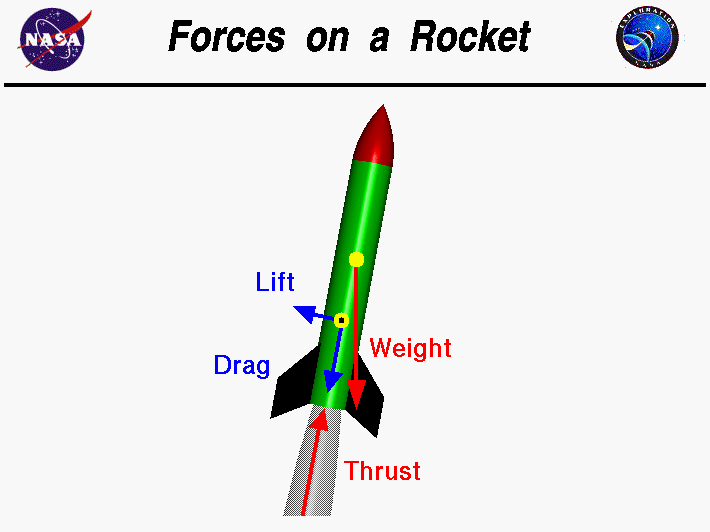# Four Forces on a RocketThe study of rockets is an excellent way for students to learn the basics of forces and the response of an object to external forces. The motion of an object in response to an external force was first accurately described over 300 years ago by Sir Isaac Newton, using his three laws of motion. Engineers still use Newton’s laws to design and predict the flight of full-scale rockets.

## Four Forces in Flight

Forces are vector quantities having both a magnitude and a direction. When describing the action of forces, one must account for both the magnitude and the direction. In flight, a rocket is subjected to four forces; weight, thrust, and the aerodynamic forces, lift and drag. The magnitude of the weight depends on the mass of all of the parts of the rocket. The weight force is always directed towards the center of the earth and acts through the center of gravity, the yellow dot on the figure. The magnitude of the thrust depends on the mass flow rate through the engine and the velocity and pressure at the exit of the nozzle. The thrust force normally acts along the longitudinal axis of the rocket and therefore acts through the center of gravity. Some full scale rockets can move, or gimbal, their nozzles to produce a force which is not aligned with the center of gravity. The resulting torque about the center of gravity can be used to maneuver the rocket.

The magnitude of the aerodynamic forces depends on the shape, size, and velocity of the rocket and on properties of the atmosphere. The aerodynamic forces act through the center of pressure, the black and yellow dot on the figure. Aerodynamic forces are very important for model rockets but may not be as important for full scale rockets, depending on the mission of the rocket. Full scale boosters usually spend only a short amount of time in the atmosphere.

## External Factors

In flight, the magnitude, and sometimes the direction, of the four forces is constantly changing. The response of the rocket depends on the relative magnitude and direction of the forces, much like the motion of the rope in a “tug-of-war” contest. If we add up the forces, being careful to account for the direction, we obtain a net external force on the rocket. The resulting motion of the rocket is described by Newton’s laws of motion.

## Differences in the Application of the Forces

Although the same four forces act on a rocket as on an airplane, there are some important differences in the application of the forces:

1. On an airplane, the lift force (the aerodynamic force perpendicular to the flight direction) is used to overcome the weight. On a rocket, thrust is used in opposition to weight. On many rockets, lift is used to stabilize and control the direction of flight.
2. On an airplane, most of the aerodynamic forces are generated by the wings and the tail surfaces. For a rocket, the aerodynamic forces are generated by the fins, nose cone, and body tube. For both airplanes and rockets, the aerodynamic forces act through the center of pressure (the yellow dot with the black center on the figure). The weight acts through the center of gravity (the yellow dot on the figure).
3. While most airplanes have a high lift to drag ratio, the drag of a rocket is usually much greater than the lift.
4. While the magnitude and direction of the forces remain fairly constant for an airplane, the magnitude and direction of the forces acting on a rocket change dramatically during a typical flight.
Provide feedback